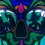# Division by 0

Lets start the discussion fresh. Some say $\frac{0}{0}$ is 0 and others 1 and most undefined.

Me on the neither side. But will start with a simple elementary proof that \frac{0}(0}=0

$\frac{0}{0} = 0\times\frac{1}{0} \\ = 0 \text{ (Since, 0 * n = 0)}$

Can you guys think of other way than usual? The usual proof is being for undetermined. What about for '1'?

Other proofs include theoretical practical sense.

We give "nothing" the value of '0'. Division represents how many of x in m, for x÷m.

Logically, for 0÷0 := How many 'nothing' in 'nothing'. Practically 'nothing', of-course! So 0 again

n÷0 := How many something in nothing. There is nothing so how can there be something, so answer is nothing =: 0Note by Viki Zeta
3 years, 11 months ago

This discussion board is a place to discuss our Daily Challenges and the math and science related to those challenges. Explanations are more than just a solution — they should explain the steps and thinking strategies that you used to obtain the solution. Comments should further the discussion of math and science.

When posting on Brilliant:

• Use the emojis to react to an explanation, whether you're congratulating a job well done , or just really confused .
• Ask specific questions about the challenge or the steps in somebody's explanation. Well-posed questions can add a lot to the discussion, but posting "I don't understand!" doesn't help anyone.
• Try to contribute something new to the discussion, whether it is an extension, generalization or other idea related to the challenge.

MarkdownAppears as
*italics* or _italics_ italics
**bold** or __bold__ bold
- bulleted- list
• bulleted
• list
1. numbered2. list
1. numbered
2. list
Note: you must add a full line of space before and after lists for them to show up correctly
paragraph 1paragraph 2

paragraph 1

paragraph 2

[example link](https://brilliant.org)example link
> This is a quote
This is a quote
    # I indented these lines
# 4 spaces, and now they show
# up as a code block.

print "hello world"
# I indented these lines
# 4 spaces, and now they show
# up as a code block.

print "hello world"
MathAppears as
Remember to wrap math in $$ ... $$ or $ ... $ to ensure proper formatting.
2 \times 3 $2 \times 3$
2^{34} $2^{34}$
a_{i-1} $a_{i-1}$
\frac{2}{3} $\frac{2}{3}$
\sqrt{2} $\sqrt{2}$
\sum_{i=1}^3 $\sum_{i=1}^3$
\sin \theta $\sin \theta$
\boxed{123} $\boxed{123}$

Sort by:

Check out what is 0 divided by 0?

Staff - 3 years, 11 months ago

Here is some cool stuff where undefined objects have been discussed to some extent.

- 3 years, 11 months ago

Uhh... $n \in \mathbb{R} \implies 0 \times n =0$. Note that $\frac{1}{0}$ is not a real number!

- 3 years, 11 months ago